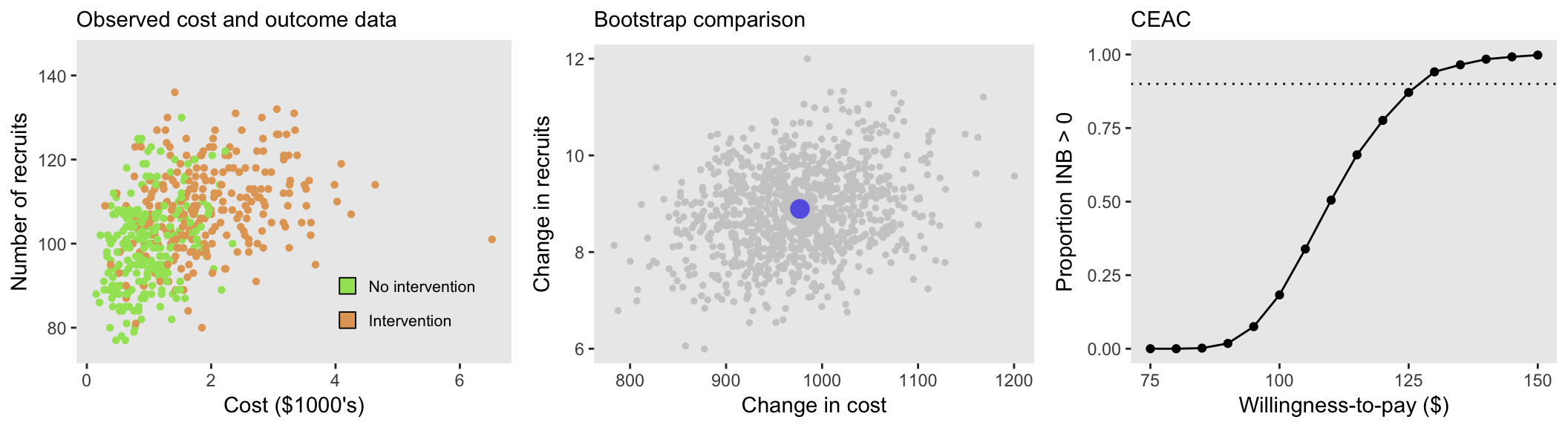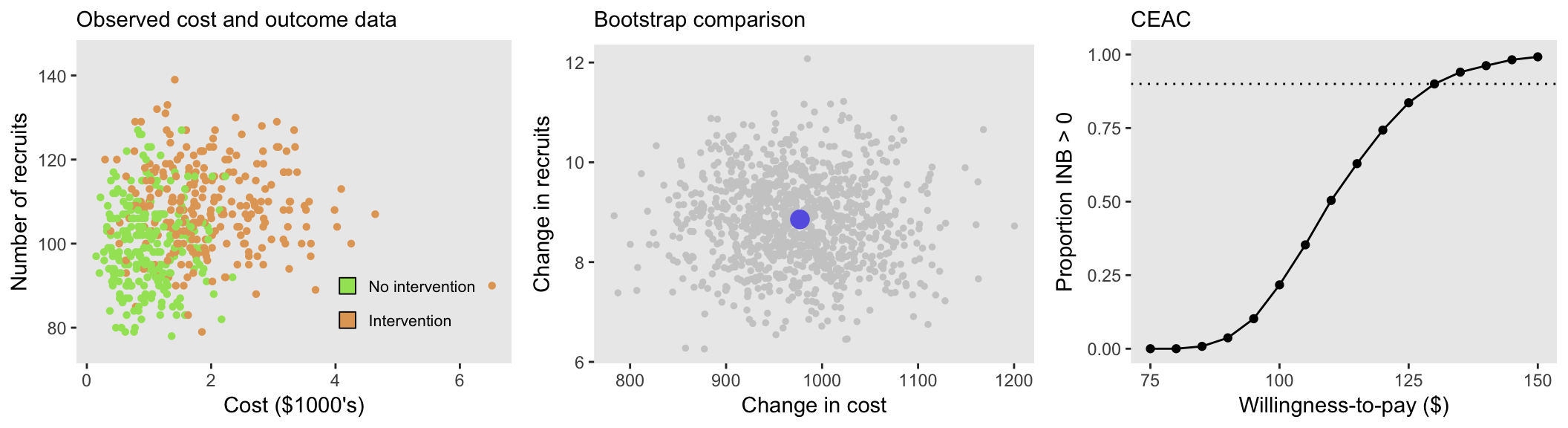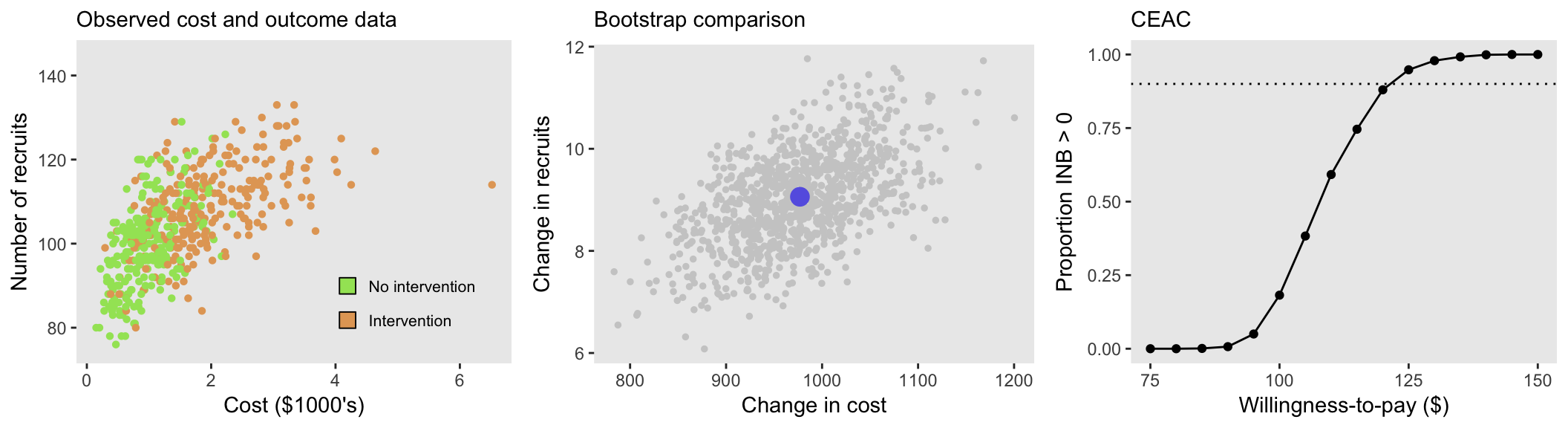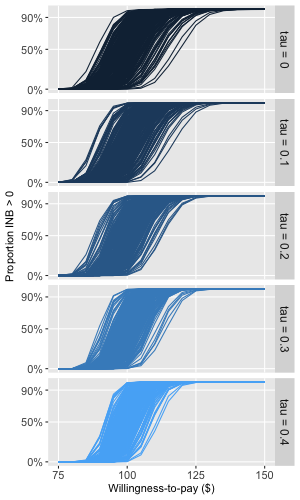My dissertation work (which I only recently completed - in 2012 - even though I am not exactly young, a whole story on its own) focused on inverse probability weighting methods to estimate a causal cost-effectiveness model. I don’t really do any cost-effectiveness analysis (CEA) anymore, but it came up very recently when some folks in the Netherlands contacted me about using simstudy to generate correlated (and clustered) data to compare different approaches to estimating cost-effectiveness. As part of this effort, I developed two more functions in simstudy that allow users to generate correlated data drawn from different types of distributions. Earlier I had created the CorGen functions to generate multivariate data from a single distribution – e.g. multivariate gamma. Now, with the new CorFlex functions (genCorFlex and addCorFlex), users can mix and match distributions. The new version of simstudy is not yet up on CRAN, but is available for download from my github site. If you use RStudio, you can install using devtools::install.github("kgoldfeld/simstudy"). [Update: simstudy version 0.1.8 is now available on CRAN.]

I thought I’d introduce this new functionality by generating some correlated cost and outcome data, and show how to estimate a cost-effectiveness analysis curve (CEAC). The CEAC is based on a measure called the incremental net benefit (INB). It is far more common in cost-effectiveness analysis to measure the incremental cost-effectiveness ratio (ICER). I was never enamored of ICERs, because ratios can behave poorly when denominators (in this case the changes in outcomes) get very small. Since it is a difference, the INB behaves much better. Furthermore, it seems relatively intuitive that a negative INB is not a good thing (i.e., it is not good if costs are greater than benefits), but a negative ICER has an unclear interpretation. My goal isn’t to give you a full explanation of CEA, but to provide an application to demonstrate the new simstudy functions. If you really want to learn more about this topic, you can find a paper here that described my dissertation work. Of course, this is a well-established field of study, so naturally there is much more out there…

### Simulation scenario

In the simulation scenario I’ve concocted, the goal is to increase the number of patients that come in for an important test. A group of public health professionals have developed a new outreach program that they think will be able to draw in more patients. The study is conducted at the site level - some sites will implement the new approach, and the others, serving as controls, will continue with the existing approach. The cost for the new approach is expected to be higher, and will vary by site. In the first scenario, we assume that costs and recruitment are correlated with each other. That is, sites that tend to spend more generally have higher recruitment levels, even before introducing the new recruitment method.

The data are simulated using the assumption that costs have a gamma distribution (since costs are positive, continuous and skewed to the right) and that recruitment numbers are Poisson distributed (since they are non-negative counts). The intervention sites will have costs that are on average $1000 greater than the control sites. Recruitment will be 10 patients higher for the intervention sites. This is an average expenditure of$100 per additional patient recruited:

library(simstudy)

# Total of 500 sites, 250 control/250 intervention

set.seed(2018)
dt <- genData(500)
dt <- trtAssign(dtName = dt, nTrt = 2,
balanced = TRUE, grpName = "trtSite")

### Cost-effectiveness: INB & the CEAC

I alluded to the fact that I believe that the incremental net benefit (INB) might be a preferable way to measure cost-effectiveness, just because the measure is more stable and easier to interpret. This is how it is defined:

$INB = \lambda (\bar{R}_{intervention} - \bar{R}_{control}) - (\bar{C}_{intervention} - \bar{C}_{control})$

where $$\lambda$$ is the willingness-to-pay I mentioned above. One of the advantages to using the INB is that we don’t need to specify $$\lambda$$, but can estimate a range of INBs based on a range of willingness-to-pay values. For all values of $$\lambda$$ where the INB exceeds $0, the intervention is cost-effective. The CEAC is a graphical approach to cost-effectiveness analysis that takes into consideration uncertainty. We estimate uncertainty using a bootstrap approach, which entails sampling repeatedly from the original “observed” data set with replacement. Each time we draw a sample, we estimate the mean differences in cost and recruitment for the two treatment arms. A plot of these estimated means gives a sense of the variability of our estimates (and we can see how strongly these means are correlated). Once we have all these bootstrapped means, we can calculate a range of INB’s for each pair of means and a range of $$\lambda$$’s. The CEAC represents the proportion of bootstrapped estimates with a positive INB at a particular level of $$\lambda$$. This is much easier to see in action. To implement this, I wrote a little function that randomly samples the original data set and estimates the means: estMeans <- function(dt, grp, boot = FALSE) { dGrp <- dt[trtSite == grp] if (boot) { size <- nrow(dGrp) bootIds <- dGrp[, sample(id, size = size, replace = TRUE)] dGrp <- dt[bootIds] } dGrp[, .(mC = mean(cost), mN = mean(nRecruits))] } First, we calculate the differences in means of the observed data: (estResult <- estMeans(dOutcomes, 1) - estMeans(dOutcomes, 0)) ## mC mN ## 1: 976.9235 8.892 Next, we draw 1000 bootstrap samples: bootResults <- data.table() for (i in 1:1000) { changes <- estMeans(dOutcomes, 1, boot = TRUE) - estMeans(dOutcomes, 0, boot = TRUE) bootResults <- rbind(bootResults, changes) } bootResults ## mC mN ## 1: 971.3087 9.784 ## 2: 953.2996 8.504 ## 3: 1053.0340 9.152 ## 4: 849.5292 8.992 ## 5: 1008.9378 8.452 ## --- ## 996: 894.0251 8.116 ## 997: 1002.0393 7.948 ## 998: 981.6729 8.784 ## 999: 1109.8255 9.596 ## 1000: 995.6786 8.736 Finally, we calculate the proportion of INBs that exceed zero for a range of $$\lambda$$’s from$75 to $150. We can see that at willingness-to-pay levels higher than$125, there is a very high probability (~90%) of the intervention being cost-effective. (At the ICER level of $110, the probability of cost-effectiveness is only around 50%.) CEAC <- data.table() for (wtp in seq(75, 150, 5)) { propPos <- bootResults[, mean((wtp * mN - mC) > 0)] CEAC <- rbind(CEAC, data.table(wtp, propPos)) } CEAC ## wtp propPos ## 1: 75 0.000 ## 2: 80 0.000 ## 3: 85 0.002 ## 4: 90 0.018 ## 5: 95 0.075 ## 6: 100 0.183 ## 7: 105 0.339 ## 8: 110 0.505 ## 9: 115 0.659 ## 10: 120 0.776 ## 11: 125 0.871 ## 12: 130 0.941 ## 13: 135 0.965 ## 14: 140 0.984 ## 15: 145 0.992 ## 16: 150 0.998 ### A visual CEA Here are three series of plots, shown for different levels of correlation between cost and recruitment. Each series includes a plot of the original cost and recruitment data, where each point represents a site. The second plot shows the average difference in means between the intervention and control sites in purple and the bootstrapped differences in grey. The third plot is the CEAC with a horizontal line drawn at 90%. The first series is the data set we generated with tau = 0.2:When there is no correlation between costs and recruitment across sites (tau = 0):And finally - when there is a higher degree of correlation, tau = 0.4:### Effect of correlation? In all three scenarios (with different levels of tau), the ICER is approximately$110. Of course, this is directly related to the fact that the estimated differences in means of the two intervention groups is the same across the scenarios. But, when we look at the three site-level and bootstrap plots, we can see the varying levels of correlation.

And while there also appears to be a subtle visual difference between the CEAC’s for different levels of correlation, it is not clear if this is a real difference or random variation. To explore this a bit further, I generated 250 data sets and their associated CEACs (which in turn are generated by 1000 bootstrap steps eacj) under a range of tau’s, starting with no correlation (tau = 0) up to a considerable level of correlation (tau = 0.4). In these simulations, I used a larger sample size of 2000 sites to reduce the variation a bit. Here are the results:It appears that the variability of the CEAC curves decreases as correlation between cost and recruitment (determined by tau) increases; the range of the curves is smallest when tau is 0.4. In addition, in looks like the “median” CEAC moves slightly rightward as tau increases, which suggests that probability of cost-effectiveness will vary across different levels of tau. All this is to say that correlation appears to matter, so it might be an important factor to consider when both simulating these sorts of data and actually conducting a CEA.

### Next steps?

In this example, I based the entire analysis on a simple non-parametric estimate of the means. In the future, I might explore copula-based methods to fit joint models of costs and outcomes. In simstudy, a Gaussian copula generates the correlated data. However there is a much larger world of copulas out there that can be used to model correlation between measures regardless of their marginal distributions. And some of these methods have been applied in the context of CEA. Stay tuned on this front (though it might be a while).